# 2.4 Phylogenetic reconstruction ¶

In this chapter we'll begin to explore the goals, approaches, and challenges for creating phylogenetic trees, or phylogenies. Phylogenies, such as the two presented in Figure 1, represent hypotheses about the evolutionary history of a group of individuals, who are represented by the tips in the tree. You can explore an interactive version of the three-domain tree presented in Figure 1b online, through the Interactive Tree of Life project.Figure 1a: Evolutionary tree presented by Charles Darwin in On the Origin of Species. Figure 1b: A hypothesis of evolutionary relationships between the three domains of life. This image was created by the Norman Pace Laboratory.

## 2.4.1 Why build phylogenies?¶

Reconstructing the phylogeny of a group of individuals is useful for many reasons. Probably the most obvious of these is understanding the evolutionary relationship between a group of organisms. For example, over the past half-century we've gained great insight into the evolution of our species, Homo sapiens, by studying features of our closest relatives, both extant (still existing) organisms, such as the Pan (chimpanzees) and Gorilla genera, and extinct (no longer living) species, including Homo neanderthalensis, Homo erectus, Homo habilus, and many species in the Australopithecus genus. (The Smithsonian Museum's Human Origins Initiative is an excellent resource for learning more about the fascinating subject of human evolution.) In this time, we've also improved our understanding of the deeper branches in the tree of life. For example, Woese and Fox (1977) used phylogenetic reconstruction to first illustrate that the "prokaryotes" really represented two ancient lineages which they called the eubacteria and the archaebacteria, which ultimately led to the proposal of a "three domain" tree of life composed of the three deep branching lineages, the archaea, the bacteria, and the eucarya (Woese, Kandler and Wheelis (1990)).

Phylogenetic trees such as these are also useful for understanding evolution itself. In fact, they're so useful that the single image that Charles Darwin found important enough to include in On the Origin of Species was the phylogenetic tree presented in Figure 1a.

The individuals represented at the tips of our trees don't necessarily have to be organisms though. In another important application of phylogenetic trees, we can study the evolution of genes, which can help us gain a deeper understanding of gene function. Specifically, we can learn about families of related genes. A classic example of this is the globin family, which includes the proteins hemoglobin and myoglobin, molecules that can reversibly bind oxygen (meaning they can bind to it, and then let go of it). You've probably heard of hemoglobin (if not globins in general), as this molecule binds to oxygen where it is present in high concentration (such as in your lung) and releases it where it is present in low concentration (such as in the bicep, where it is ultimately used to power your arm). Hemoglobin and myoglobin are paralogs, meaning that they are related by a gene duplication and subsequent divergence. If you were to compare an unknown globin sequence to either of these you could detect homology, but a tree such as the one present in Figure 2, would help you understand the type of homologous relationship (i.e., whether it was orthology or paralogy).Figure 2: A tree representing members of the globin gene family from diverse taxa. This image is an unmodified reproduction of Figure 5 from A phylogenomic profile of globins by Vinogradov et al (2006).

Phylogenetic trees are used for many other diverse applications in bioinformatics, so it's therefore important that a bioinformatician have an understanding of how they are built and how they should be interpreted. An additional application that we'll cover in this text is comparing the composition of communities of organisms, but we'll come back to that later.

## 2.4.2 How phylogenies are reconstructed¶

Phylogenies are reconstructed using a variety of different algorithms, some of which we'll cover in this chapter. These algorithms all work by comparing a set of features of organisms, and inferring the evolutionary distance between those organisms based on the similarity of their features. The features that are compared can be nearly anything that is observable, either from extant organisms or fossilized representatives of extinct organisms.

As an example, let's consider the reconstruction of the phylogeny of spiders (the order Araneae), a hypothesis of which is presented in Figure 3. Of the extant spiders, some are orb-weavers (meaning they spin circular, sticky webs), and others are not. Entomologists have debated whether orb-weaving is a monophyletic trait (meaning that it evolved one time), or whether it is polyphyletic (meaning that it evolved multiple times, such as flight, which has evolved independently in birds, flying dinosaurs, insects, and mammals). If orb-weaving is monophyletic, it would mean that over the course of evolution, extant spiders which don't weave orb webs have lost that ability. Some researchers doubt this as it's a very effective means of catching prey, and losing that ability would likely constitute an evolutionary disadvantage. If orb-weaving is polyphyletic, it would means that in at least two different spider lineages, this trait arose independently, which other researchers consider to be very unlikely due to the complexity of engineering these webs. Examples of the evolution of monophyletic and polyphyletic traits are presented in Figures 3 and 4, respectively.Figure 3: Example phylogeny illustrating a monophyletic trait shared by a group of organisms. In a monophyletic group, the last common ancestor was also member of the group (e.g., multicellular organisms).Figure 4: Example phylogeny illustrating a polyphyletic trait shared by a group of organisms. In a polyphyletic group the last common ancestor was not a member of the group (e.g., flying animals).

Earlier work on understanding the relations between the spider lineages focused on comparing traits that entomologists would observe, for example by watching spiders in action or by dissecting them. For example, in 1986 through 1991, Johnathan Coddington published several studies that tabulated and compared about sixty features of 32 spider taxa (Coddington J. 1986. The monophyletic origin of the orb web. In: Shear W, ed. Spiders: webs, behavior, and evolution. Stanford, California: Stanford University Press. 319-363; Coddington JA. 1991. Cladistics and spider classification: araneomorph phylogeny and the monophyly of orbweavers (Araneae: Araneomorphae; Orbiculariae) Acta Zoologica Fennica 190:75-87). Features included whether a spider wraps its prey when it attacks (a behavioral trait), and how branched the spider's trachea is (a morphological trait). By determining which spiders were more similar and different across these traits, Dr. Coddington provided early evidence for the hypothesis that orb-weaving is an ancient monophyletic trait.

More recently, several research teams have used features of spider genomes to reconstruct the spider phylogeny (Bond et al., 2014, Garrison et al., 2016). Using this approach, the features become the nucleotides observed at particular positions in the genome, which are observed first by sequencing specific genes that the researchers target that are present in all members of the group, and then aligning those sequences with multiple sequence alignment. This has several advantages over feature matrices derived from morphological and behavioral traits, including that many more features can be observed. For example, (Garrison et al., 2016), compared approximately 700,000 amino acid positions from nearly 4000 loci around the genomes of 70 spider taxa. Compare the number of features here to the number mentioned in the previous paragraph. These phylogenomic studies have further supported the idea that orb-weaving is an ancient monophyletic trait, and have provided much finer scale information on the evolution of spiders. Supported by these data, researchers hypothesize that the loss of orb-weaving might not be that surprising. While it does provide an effective means of catching flying insects, many insects which are potential prey for spiders don't fly. Further, orb webs may attract predators of spiders, as they are easily observable signals of where a spider can be found.Figure 5: A spider phylogeny. Numbers at internal nodes correspond to the taxonomic groups described in Table 1 of Garrison et al., 2016. This image is an unmodified version of Figure 1 of Garrison et al., 2016.

For the remainder of this chapter, we'll consider methods for phylogenetic reconstruction that use genome sequence data as features. ## [2.4.3](#3) Some terminology  Next, let's cover a few terms using the tree diagram in Figure 6.Figure 6: A schematic of a phylogenetic tree illustrating important terms.

Terminal nodes or tips typically represent extant organisms, also frequently called operational taxonomic units or OTUs. OTU is a generic way of referring to a grouping of organisms (such as a species, a genus, or a phylum), without specifically identifying what that grouping is.

Internal nodes in a phylogenetic tree represent hypothetical ancestors. We postulate their existence but often don't have direct evidence. The root node is the internal node from which all other nodes in the tree descend. This is often referred to as the last common ancestor (LCA) of the OTUs represented in the tree. In a universal tree of life, the LCA is often referred to as LUCA, the last universal common ancestor. All nodes in the tree can be referred to as OTUs.

Branches connect the nodes in the tree, and generally represent time or some amount of evolutionary change between the OTUs. The specific meaning of the branches will be dependent on the method that was used to build the phylogenetic tree.

A clade in a tree refers to some node (either internal or terminal) and all nodes descending from it (i.e., moving away from the root toward the tips).

We'll use all of these terms below as we begin to explore phylogenetic trees.

## 2.4.4 Simulating evolution¶

Table of Contents

Before we jump into how to reconstruct a phylogeny from DNA sequence data, we're going to perform a simulation of the process of evolution of a DNA sequence. In this simulation, we're going to model sequence evolution with a Python function, and then we're going to run that function to simulate multiple generations of evolution.

Bioinformatics developers often use simulations to understand how their algorithms work, as they uniquely provide an opportunity to know what the correct answer is. This provides a way to compare algorithms to each other to figure out which performs best under which circumstances. In our simulation we're going to have control over the starting sequence, and the probability of incurring a substitution mutation or an insertion/deletion mutation at each position of the sequence in each generation. This would, for example, let us understand whether different algorithms for phylogenetic reconstruction are better or worse for more closely or distantly related sequences.

Our simulation will works as follows. We'll have one function that we primarily interact with called evolve_generations. This will take a starting sequence and the number of generations that we want to simulate. It will also take the probability that we want a substitution mutation to occur at each position in the starting sequence, and the probability that we want either an insertion or deletion mutation to occur at each position. In each generation, every sequence will spawn two new sequences, randomly incurring mutations at the prescribed rates. This effectively simulates a clonal process of reproduction, a form of asexual reproduction common in single cellular organisms, where a parent cell divides into two cells, each containing a copy of the parent's genome with some errors introduced.

Let's inspect this code and then run our simulation beginning with a random sequence.

In :
%pylab inline

import numpy as np
import seaborn as sns
import random

from IPython.core import page
page.page = print

Populating the interactive namespace from numpy and matplotlib


First we'll look at the function used to simulate the evolution of a single sequence. This is where most of the important evolutionary modeling of sequence evolution happens.

In :
from iab.algorithms import evolve_sequence
%psource evolve_sequence

def evolve_sequence(sequence, substitution_probability, indel_probability):
result = []
sequence_length = len(sequence)
insertion_choices = list(sequence.nondegenerate_chars)

result = []
i = 0
while i < sequence_length:
current_char = sequence[i]
if random.random() < substitution_probability:
# simulate a substitution event by adding a character other than the current
# character to the result at this position
substituted_base = random.choice([r for r in sequence.nondegenerate_chars if r != current_char])
result.append(substituted_base)
i += 1
elif random.random() < indel_probability:
# simulate either an insertion or a deletion event. the length of the insertion or
# deletion is determined at random, with shorter lengths being more probable
length = int(np.random.triangular(1, 1, 10))
if np.random.binomial(1, 0.5) == 0:
# simulate an insertion by adding length random characters from
# this sequence's alphabet
result.extend(np.random.choice(insertion_choices, size=length))
i += 1
else:
# simulate a deletion by not appending any of the next length
# characters
i += length
else:
# simulate no mutation occurring
result.append(str(current_char))
i += 1

return sequence.__class__(''.join(result))



Next, take a look at the function that models a single generation of a single sequence. This is where the clonal reproduction (i.e., one parent sequence becoming two child sequences) occurs.

In :
from iab.algorithms import evolve_generation
%psource evolve_generation

def evolve_generation(sequence,
substitution_probability,
indel_probability,
increased_rate_probability,
fold_rate_increase):
child1 = evolve_sequence(sequence, substitution_probability, indel_probability)
if random.random() < increased_rate_probability:
child2 = evolve_sequence(sequence, fold_rate_increase * substitution_probability, indel_probability)
else:
child2 = evolve_sequence(sequence, substitution_probability, indel_probability)
return child1, child2



Finally, take a look at our entry point function. This is where we provide the parameters of our simulation, including the starting sequence, the number of generations, and the mutation probabilities. Notice how each of these functions builds on the prior functions.

In :
from iab.algorithms import evolve_generations
%psource evolve_generations

def evolve_generations(ancestral_sequence, generations, substitution_probability,
indel_probability, increased_rate_probability=0.0,
fold_rate_increase=5, verbose=False):
"""
ancestral_sequence : skbio.Sequence object
The sequence that should be used as the root node in the tree.
generations : int
The number of generations to simulate. Must be greater than zero.
substitution_probability : float
The probability at which a substitution mutation should occur.
indel_probability : float
The probability at which either an insertion or a deletion mutation
should occur. One of these will be simulated with even probability,
and the length will be randomly chosen between 1 and 9, with shorter
lengths being more probable.
increased_rate_probability : float, optional
The probability at which child2 should experience an increased rate
of substitution mutations. Default is that this never occurs.
fold_rate_increase : int, optional
If increased_rate_probability is greater than zero, the fold increase
that substitutions should now occur at.
verbose : bool, optional
If True, print the sequences that are simulated at each generation.

"""
# initial some values and perform some basic error checking
assert generations > 0, "Must simulate one or more generations."

# initialize a list of the previous generations sequences - this gets used
# in the for loop below. since we'll start with the first generation of
# children, root_sequence is the previous generation's sequence
ancestral_sequence.metadata['id'] = '0'
previous_generation_sequences = [ancestral_sequence]

# iterate for each requested generation
for i in range(generations):
# print the generation number and the current number of sequences
if verbose:
print("Generation: %d (Number of parent sequences: %d)" % (i,2**i))
print("%s (last common ancestor)" % ancestral_sequence)
print("")

# create a list to store the current generation of sequences
current_generation_sequences = []

# iterate over the sequences of the previous generation
for parent_sequence in previous_generation_sequences:
# evolve two child sequences - currently the mutation probabilities are
# constant, but should update that to change with generations
r1, r2 = evolve_generation(parent_sequence, substitution_probability, indel_probability,
increased_rate_probability, fold_rate_increase)
r1.metadata['id'] = '%s.1' % (parent_sequence.metadata['id'])
r2.metadata['id'] = '%s.2' % (parent_sequence.metadata['id'])
current_generation_sequences.extend([r1, r2])
if verbose:
# if the caller specified verbose output, print the actual sequences
print("%s (parent id: %s)" % (parent_sequence, parent_sequence.metadata['id']))
print("%s (child 1 id: %s)" % (r1, r1.metadata['id']))
print("%s (child 2 id: %s)" % (r2, r2.metadata['id']))
print("")
# current_generation_sequences becomes the next generation's
# previous_generation_sequences
previous_generation_sequences = current_generation_sequences

# upon completion of all generations, return the last generation's sequences
return previous_generation_sequences



Now we'll run our simulation. We'll start with a random DNA sequence, and then evolve three generations. Before running this, can you predict how many child sequences we'll end up with after three generations?

When we call evolve_generations, we'll pass the parameter verbose=True. This will tell the function to print out some information throughout the process. This will let us inspect our evolutionary process: something that is impossible to do with real sequences.

In :
from iab.algorithms import random_sequence
import skbio
sequence = random_sequence(skbio.DNA, 50)

In :
sequences = evolve_generations(sequence, generations=3, substitution_probability=0.1, indel_probability=0.05,
increased_rate_probability=0.1, verbose=True)

Generation: 0 (Number of parent sequences: 1)
GGATCGCATGTGGATGTCTCCGCATACGTTCTCAGTATATCGTCGAAATA (last common ancestor)

GGATCGCATGTGGATGTCTCCGCATACGTTCTCAGTATATCGTCGAAATA (parent id: 0)
TCGCACCAGTGACTGTCCGCATACGTTCGCAGTATATCGTCGAAATA (child 1 id: 0.1)
GGATCGGTTGTGGATGTCTCCGCAACGTTCTCAGTCTAGCGGAAATA (child 2 id: 0.2)

Generation: 1 (Number of parent sequences: 2)
GGATCGCATGTGGATGTCTCCGCATACGTTCTCAGTATATCGTCGAAATA (last common ancestor)

TCGCACCAGTGACTGTCCGCATACGTTCGCAGTATATCGTCGAAATA (parent id: 0.1)
TCGCACCAGTGACTGTCGGTATATGTTCGCACTATATCGTCGCAATA (child 1 id: 0.1.1)
TAGTGACTGTCCGCATACTTTCGCAGTATATCGTCGAAATA (child 2 id: 0.1.2)

GGATCGGTTGTGGATGTCTCCGCAACGTTCTCAGTCTAGCGGAAATA (parent id: 0.2)
GGATCAGTTGTGGTCCGGCACGTTCGCAGTCTACCAAATA (child 1 id: 0.2.1)
GGATCGCTTGTGATGTTCCGCAAGTTAATTTCAGTCTAGCGGAAATA (child 2 id: 0.2.2)

Generation: 2 (Number of parent sequences: 4)
GGATCGCATGTGGATGTCTCCGCATACGTTCTCAGTATATCGTCGAAATA (last common ancestor)

TCGCACCAGTGACTGTCGGTATATGTTCGCACTATATCGTCGCAATA (parent id: 0.1.1)
TCGCAGTGACTACCATGTTCGCACTATACCATCGCAATA (child 1 id: 0.1.1.1)
TCGCCCCAGGAACTGTCGGTATATGATCGCACGTCGCAATA (child 2 id: 0.1.1.2)

TAGTGACTGTCCGCATACTTTCGCAGTATATCGTCGAAATA (parent id: 0.1.2)
TAGTGAGAAGTGTATGTCCGCATACTTTCGCGGTATATCGTCAACATA (child 1 id: 0.1.2.1)
TAGTGACTGTCCGTATAGTTTGCGGCAGTATATCGTCGAAACCTA (child 2 id: 0.1.2.2)

GGATCAGTTGTGGTCCGGCACGTTCGCAGTCTACCAAATA (parent id: 0.2.1)
GGATCAGTTGTGGTCCTGCACGTTCCCAGGCTACCCGGTTAATA (child 1 id: 0.2.1.1)
GGATCCGTTGTGGTCCGGCACGTTCGCAGTCTACCAAATA (child 2 id: 0.2.1.2)

GGATCGCTTGTGATGTTCCGCAAGTTAATTTCAGTCTAGCGGAAATA (parent id: 0.2.2)
GGATCGCTTGTGATGTTACGCACGTTAATATCAGACTAGCGGAAATA (child 1 id: 0.2.2.1)
TGAACGAGGCTTTCCTGTTCCGTGAGGTGCTTTAATTCAAGACGAATTA (child 2 id: 0.2.2.2)



We now have a new variable, sequences, which contains the child sequences from the last generation. Take a minute to look at the ids of the parent and child sequences above, and the ids of a couple of the final generation sequences. These ids are constructed so that each sequence contains the identifiers of its ancestral sequences, and then either 1 or 2. Notice that all sequence identifiers start with 0, the identifier of the last common ancestor (or our starting sequence) of all of the sequences. These identifiers will help us interpret whether the phylogenies that we reconstruct accurately represent the evolutionary relationships between the sequences.

Also, notice that at this point we only have the sequences from the last generation. We no longer have the ancestral sequences (which would correspond to the internal nodes in the tree). This models the real world, were we only have sequences from extant organisms, but not their ancestors.

Take a minute to compare the two sequences below. What types of mutations happened over the course of their evolution?

In :
print(len(sequences))

8

In :
sequences

Out:
DNA
--------------------------------------------
Metadata:
'id': '0.1.1.1'
Stats:
length: 39
has gaps: False
has degenerates: False
has non-degenerates: True
GC-content: 46.15%
--------------------------------------------
0 TCGCAGTGAC TACCATGTTC GCACTATACC ATCGCAATA
In :
sequences[-1]

Out:
DNA
-------------------------------------------------------
Metadata:
'id': '0.2.2.2'
Stats:
length: 49
has gaps: False
has degenerates: False
has non-degenerates: True
GC-content: 42.86%
-------------------------------------------------------
0 TGAACGAGGC TTTCCTGTTC CGTGAGGTGC TTTAATTCAA GACGAATTA

In our simulation, each sequence is directly derived from exactly one sequence from the previous generation, and the evolution of all of the sequences traces back to starting sequence that we provided. This means that our final sequences are all homologous. And because we have modeled this process, we know where each sequence fits in relation to all of the other sequences in the phylogeny. Our goal with the algorithms we'll study for the rest of this chapter is to reconstruct that phylogeny given only the last generation of sequences. We'll use the fact that we know the true phylogeny to help us evaluate the relative performance of the different methods.Figure 7: Schematic of a simulated evolutionary process. Bases in red indicate mutation since the last common ancestor. The bottom panel illustrates the real-world equivalent of our final product, where we wouldn't know the true phylogeny (indicated by the dashed branches), the sequence of the last common ancestor, or what positions have changed since the last common ancestor.

Let's simulate 10 generations of sequences here, and then randomly select some of those sequences to work with in the remaining sections of this chapter. For the sake of runtime, I'm going to set our indel_probability=0.0. This means that we won't need to align our selected sequences before constructing trees from them (because with only substitution mutations occurring, homologous positions always remain aligned). Multiple sequence alignment is currently a runtime bottleneck in IAB, so this will let us run this notebook much faster. If you'd like to model insertion/deletions, you can increase the indel_probability, say to 0.005. If you do that, the sequences will be aligned for you in the next cell, but it may take around 30 minutes to run.

In :
from skbio.alignment import global_pairwise_align_nucleotide
from iab.algorithms import progressive_msa
from functools import partial

indel_probability = 0.0

sequences = evolve_generations(sequence, generations=10, substitution_probability=0.03,
indel_probability=0.0, increased_rate_probability=0.1, verbose=False)

sequences = random.sample(sequences, 25)

if indel_probability == 0:
sequences_aligned = sequences
else:
gpa = partial(global_pairwise_align_nucleotide, penalize_terminal_gaps=True)
sequences_aligned = progressive_msa(sequences, pairwise_aligner=gpa)


### 2.4.4.1 A cautionary word about simulations¶

While simulations are extremely powerful for comparing algorithms, they can also be misleading. This is because when we model evolution we simplify the evolutionary process. One assumption that our simulation is making is that "bursts" of evolution (i.e., where our substitution rate temporarily increases) are restricted to only a single generation. A child is no more or less like to have an increased rate of substitutions if its parent did. This may or may not be an accurate model. Imagine in the real-world that the environment changed drastically for some descendants (for example, if a geological event created new thermal vents in a lake they inhabited, resulting in an increase in mean water temperature), but not for others. The lineages who experience the environmental change might have an increased rate of substitutions as their genomes adapt to the new environment. The increased substitution rate may persist for multiple generations, or it may not. This is one limitation of our simulation that we know about, but because we don't have a perfect understanding of sequence evolution, there are limitations that we don't know about.

When using simulations, it's important to understand what assumptions the simulation makes so you know what it can tell you about and what it can't tell you about. You'll want to consider that when determining how confident you are in the results of an evaluation based on simulation. What are some other assumptions that are being made by the evolutionary simulation presented here? There are many, so take a minute to list a few.

On the opposite end of the spectrum from simulations for algorithm comparison is comparisons based on real data. The trade-off is that, while there are no assumptions being made in real data with respect to the composition of the sequences, with real data we don't know what the right answer (in our case, the correct phylogeny) is, so it's harder to determine which algorithms are doing better or worse. The take-away message here is that neither approach is perfect, and often researchers will use a combination of simulated and real data to evaluate algorithms.

## 2.4.5 Visualizing trees with ete3¶

As we now start computing phylogenetic trees, we're going to need a way to visualize them. We'll use the ete3 Python package for this, and in the next cell we'll configure the TreeStyle which is used to define how the trees we visualize will look. If you'd like to experiment with other views, you can modify the code in this cell according to the ete3 documentation. If you come up with a nicer style, I'd be interested in seeing that in a pull request. You can post screenshots to IAB issue #213 before creating a pull request so I can see what the new style looks like.

In :
import ete3
ts = ete3.TreeStyle()
ts.show_leaf_name = True
ts.scale = 250
ts.branch_vertical_margin = 15


We can apply this TreeStyle to a random tree as follows. Any changes that you make to the TreeStyle above will impact this tree and all following trees in this chapter. Experiment with this - it's fun!

In :
t = ete3.Tree()
t.populate(10)
t.render("%%inline", tree_style=ts)

Out: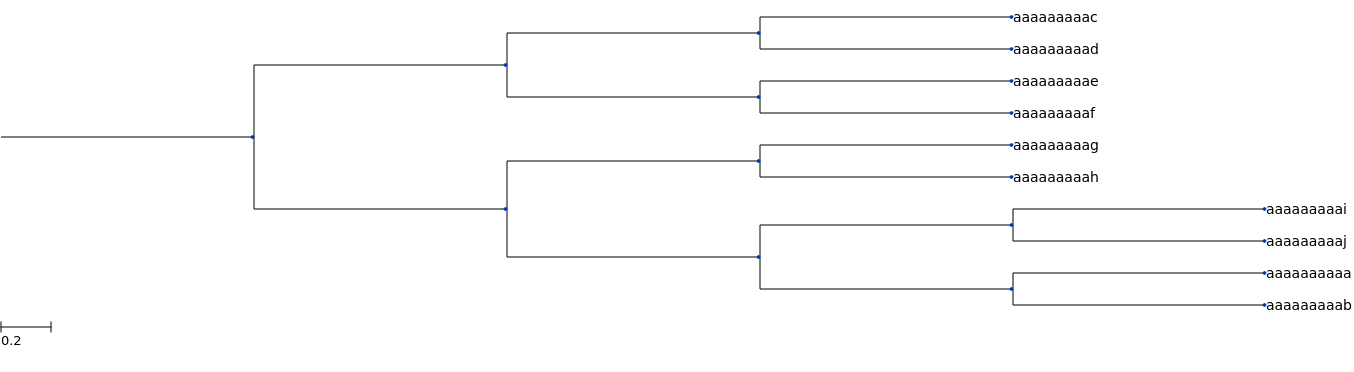## 2.4.6 Distance-based approaches to phylogenetic reconstruction¶

The next approaches we'll take for phylogenetic reconstruction rely on computing distances between sequences. We've previously discussed distances between sequences in a few places in the text. We'll begin this section by formalizing the term distance, and introducing the concept of a distance matrix.

### 2.4.6.1 Distances and distance matrices¶

Technically speaking, a distance between a pair of objects is a measure of their dissimilarity. There isn't one single definition of the distance between two objects. For example, if your two objects are the cities Flagstaff, Arizona and Boulder, Colorado you might measure the distance between them as length of shortest line that connects them. This would be the Euclidean distance between the two cities. If you're trying to travel from one city to another however, that might not be the most relevant distance measure. Instead, you might be interested in something like their Manhatten distance, which in this would be more similar to a measure of the shortest route that you could travel between the two cities using the Interstate Highway system. You can see how this is different than the shortest line connecting the two cities.

Similarly, different distance metrics will be relevant and not relevant for different types of objects. Clearly the way you measure distances between cities is very different than the way we measure distances between biological sequences. However, the underlying concept of a distance between two objects is the same.

Formally, a measure of dissimilarity $d$ between two objects $x$ and $y$ is a distance if it meets these four criteria for all $x$ and $y$:

1. $d(x,y) \geq 0$ (non-negativity)
2. $d(x,y) = 0\ if\ and\ only\ if\ x = y$ (identity of indiscernibles)
3. $d(x,y) = d(y,x)$ (symmetry)
4. $d(x,z) \leq d(x,y) + d(y,z)$ (triangle inequality)

When we compute the distances between some number of objects $n$, we'll commonly represent those values in a distance matrix which contains all of those values. These distance matrices are so common in bioinformatics that scikit-bio defines a DistanceMatrix object that provides a convenient interface for working with these data. We can create one of these objects as follows:

In :
from skbio import DistanceMatrix
dm = DistanceMatrix([[0.0, 1.0, 2.0],
[1.0, 0.0, 3.0],
[2.0, 3.0, 0.0]],
ids=['a', 'b', 'c'])


We can then access the values in the distance matrix directly, view the distance matrix as a heatmap, and do many other things that facilitate analyzing distances between objects.

In :
print(dm['a', 'b'])
print(dm['b', 'c'])

1.0
3.0

In :
_ = dm.plot(cmap='Greens')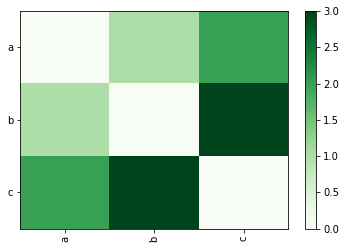The conditions of a distance metric listed above lead to a few specific features of distance matrices: they're symmetric (if you flip the upper triangle over the diagonal, the values are the same as those in the lower triangle), hollow (the diagonal is all zeros), and all values are greater than or equal to zero. Which of the conditions listed above results in each of these features of distance matrices?

### 2.4.6.2 Alignment-free distances between sequences¶

We've now looked at several ways of computing distances between sequences, some of which have required that the positions in the sequences are directly comparable to one another (i.e., that our sequences are aligned), and some of which haven't. One alignment-free distance between sequences is the k-mer distance that we worked with in Sequence Homology Searching.

We can use the kmer_distance function with scikit-bio as follows to create an skbio.DistanceMatrix object. These skbio.DistanceMatrix objects can be viewed as heatmaps. When interpreting these heatmaps, be sure to note the scale on the color bar. Since the distance metrics we compare here differ in their maximum values, the scale differs for each of these such that the darkest color can represent a different value in each heatmap.

In :
from iab.algorithms import kmer_distance
kmer_dm = DistanceMatrix.from_iterable(sequences, metric=kmer_distance, key='id')
_ = kmer_dm.plot(cmap='Greens', title='3mer distances between sequences')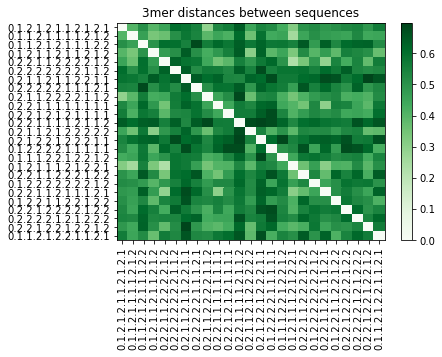### 2.4.6.3 Alignment-based distances between sequences¶

One alignment-based distance metric that we've looked at is Hamming distance. This would be considered an alignment-based approach because is does consider the order of the characters in the sequence by comparing a character at a position in one sequence only to the character at the corresponding position in the other sequence. We could compute these distances as follows, after first aligning our sequences.

In :
from skbio.sequence.distance import hamming
hamming_dm = DistanceMatrix.from_iterable(sequences_aligned, metric=hamming, key='id')
_ = hamming_dm.plot(cmap='Greens', title='Hamming distances between sequences')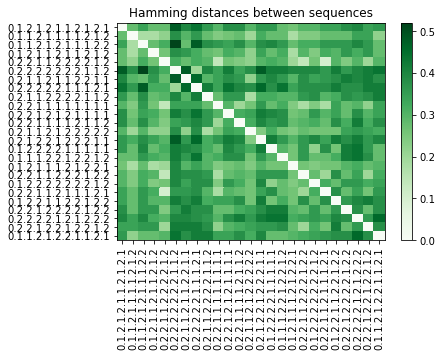### 2.4.6.4 Jukes-Cantor correction of observed distances between sequences¶

The Hamming distance between aligned sequences, as described above, is simple to calculate, but it is often an underestimate of the actual amount of mutation that has occurred in a sequence. Here's why: imagine that in one generation $g$, position $p$ of sequence $S1$ undergoes a substitution mutation from A to C. Then, in the next generation $g + 1$, the same position $p$ of sequence $S1$ undergoes a substitution from C to T. Because we can only inspect the modern-day sequences, not their ancestors, it looks like position $p$ has had a single substitution event. Similarly, if in generation $g + 1$ position $p$ changed from C back to A (a back substitution), we would observe zero substitution mutations at that position even though two had occurred.

To correct for this, the Jukes-Cantor correction is typically applied to the Hamming distances between the sequences. Where $p$ is the Hamming distance, the corrected genetic distance is computed as $d = -\frac{3}{4} \ln(1 - \frac{4}{3}p)$. The derivation of this formula is beyond the scope of this text (you can find it in Inferring Phylogeny by Felsenstein), but it is based on the Jukes-Cantor (JC69) nucleotide substitution model.

The Python implementation of this correction looks like the following. We can apply this to a number of input distance values to understand how it transforms our Hamming distances.

In :
def jc_correction(p):
return (-3/4) * np.log(1 - (4*p/3))

In :
distances = np.arange(0, 0.70, 0.05)
jc_corrected_distances = list(map(jc_correction, distances))

ax = sns.pointplot(distances, jc_corrected_distances)
ax.set_xlabel('Hamming distance')
ax.set_ylabel('JC-corrected distance')
ax.set_xlim(0)
ax.set_ylim(0)
ax

Out:
<matplotlib.axes._subplots.AxesSubplot at 0x7faee77a7ac8>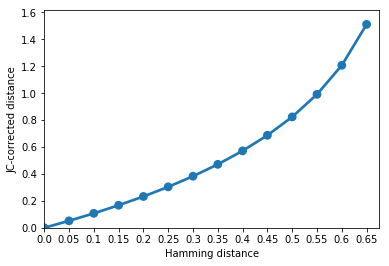We can then apply this to a full distance matrix as follows (we'll then print the first row of each).

In :
def jc_correct_dm(dm):
result = np.zeros(dm.shape)
for i in range(dm.shape):
for j in range(i):
result[i,j] = result[j,i] = jc_correction(dm[i,j])
return skbio.DistanceMatrix(result, ids=dm.ids)

jc_corrected_hamming_dm = jc_correct_dm(hamming_dm)

In :
print(hamming_dm)
print(jc_corrected_hamming_dm)

[ 0.    0.28  0.34  0.28  0.28  0.48  0.4   0.44  0.36  0.3   0.38  0.38
0.3   0.36  0.36  0.28  0.26  0.3   0.3   0.34  0.34  0.38  0.4   0.34
0.36]
[ 0.          0.35050538  0.45293704  0.35050538  0.35050538  0.76623844
0.57160504  0.66262568  0.49044485  0.38311922  0.52992765  0.52992765
0.38311922  0.49044485  0.49044485  0.35050538  0.31925086  0.38311922
0.38311922  0.45293704  0.45293704  0.52992765  0.57160504  0.45293704
0.49044485]

In :
_ = jc_corrected_hamming_dm.plot(cmap='Greens', title='JC-corrected Hamming distances between sequences')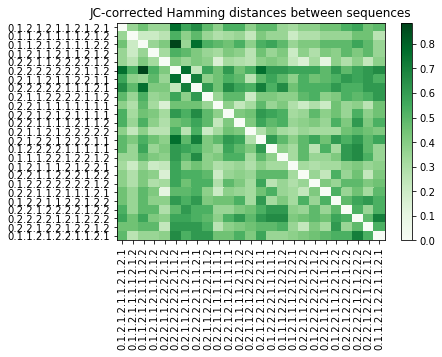### 2.4.6.5 Phylogenetic reconstruction with UPGMA¶

Table of Contents

The first algorithm we'll look at for reconstructing phylogenetic trees is called UPGMA, which stands for Unweighted Pair-Group Method with Arithmetic mean. While that name sounds complex, it's actually a straightforward algorithm, which is why we're starting with it. After we work through the algorithm, we'll come back to the name as it'll make more sense then.

UPGMA is a generic hierarchical clustering algorithm. It's not specific to reconstructing biological trees, but rather is used for interpreting any type of distance matrix. It is fairly widely used for building phylogenetic trees, though it's application in phylogenetics is usually restricted to building preliminary trees to "guide" the process of multiple sequence alignment. The reason for this is that it's fast, but it makes some assumptions that don't work well for inferring relationships between organisms, which we'll discuss after working through the algorithm.

UPGMA starts with a distance matrix, and works through the following steps to create a tree.

Step 1: Find the smallest non-zero distance in the matrix and define a clade containing only those members. Draw that clade, and set the total length of the branch connecting the tips to the distance between the tips. The distance between each tip and the node connecting them should be half of the distance between the tips.

Step 2: Create a new distance matrix with an entry representing the new clade created in step 1.

Step 3: Calculate the distance matrix entries for the new clade as the mean distance from each of the tips of the new clade to all other tips in the original distance matrix.

Step 4: If there is only one distance (below or above the diagonal) in the distance matrix, use it to connect the remaining unconnected clades, and stop. Otherwise repeat step 1.

Let's work through these steps for a simple distance matrix representing the distances between five sequences.

In :
_data = np.array([[ 0.,  4.,  2.,  5.,  6.],
[ 4.,  0.,  3.,  6.,  5.],
[ 2.,  3.,  0.,  3.,  4.],
[ 5.,  6.,  3.,  0.,  1.],
[ 6.,  5.,  4.,  1.,  0.]])
_ids = ['s1', 's2', 's3', 's4', 's5']
master_upgma_dm = skbio.DistanceMatrix(_data, _ids)
print(master_upgma_dm)

5x5 distance matrix
IDs:
's1', 's2', 's3', 's4', 's5'
Data:
[[ 0.  4.  2.  5.  6.]
[ 4.  0.  3.  6.  5.]
[ 2.  3.  0.  3.  4.]
[ 5.  6.  3.  0.  1.]
[ 6.  5.  4.  1.  0.]]


## Iteration 1¶

Step 1: The smallest non-zero distance in the above matrix is between s4 and s5. So, we'll draw that clade and set each branch length to half of the distance between them.Step 2: Next, we'll create a new, smaller distance matrix where the sequences s4 and s5 are now represented by a single clade which we'll call (s4, s5). This notation indicates that the corresponding distances are to both s4 and s5.

In :
iter1_ids = ['s1', 's2', 's3', '(s4, s5)']
iter1_dm = [[0.0,   4.0,  2.0, None],
[4.0,   0.0,  3.0, None],
[2.0,   3.0,  0.0, None],
[None, None, None, None]]


Step 3: We'll now fill in the values from the new clade to each of the existing sequences (or clades). The distance will be the mean between each pre-existing clade, and each of the sequences in the new clade. For example, the distance between s1 and (s4, s5) is the mean of the distance between s1 and s4 and s1 and s5:

In :
import numpy as np

s1_s4s5 = np.mean([master_upgma_dm['s1', 's4'], master_upgma_dm['s1', 's5']])
print(s1_s4s5)

5.5


Similarly, the distance between s2 and (s4, s5) is the mean of the distance between s2 and s4 and s2 and s5:

In :
s2_s4s5 = np.mean([master_upgma_dm['s2', 's4'], master_upgma_dm['s2', 's5']])
print(s2_s4s5)

5.5


And finally, the distance between s3 and (s4, s5) is the mean of the distance between s3 and s4 and the distance between s3 and s5:

In :
s3_s4s5 = np.mean([master_upgma_dm['s3', 's4'], master_upgma_dm['s3', 's5']])
print(s3_s4s5)

3.5


We can fill these values in to our iteration 1 distance matrix. Why do we only need to compute three values to fill in seven cells in this distance matrix?

In :
iter1_dm = [[0.0, 4.0, 2.0, s1_s4s5],
[4.0, 0.0, 3.0, s2_s4s5],
[2.0, 3.0, 0.0, s3_s4s5],
[s1_s4s5, s2_s4s5, s3_s4s5, 0.0]]

iter1_dm = DistanceMatrix(iter1_dm, iter1_ids)
print(iter1_dm)

4x4 distance matrix
IDs:
's1', 's2', 's3', '(s4, s5)'
Data:
[[ 0.   4.   2.   5.5]
[ 4.   0.   3.   5.5]
[ 2.   3.   0.   3.5]
[ 5.5  5.5  3.5  0. ]]


Step 4: Because there is still more than one value below the diagonal in our new distance matrix, we start a new iteration by going back to Step 1 and repeating this process.

## Iteration 2¶

Step 1: The smallest non-zero distance in the iteration 1 distance matrix is between s1 and s3. So, we'll draw that clade and set each branch length to half of that distance.Step 2: We next create a new, smaller distance matrix where the sequences s1 and s3 are now represented by a single clade, (s1, s3).

In :
iter2_ids = ['(s1, s3)', 's2', '(s4, s5)']
iter2_dm = [[None, None, None],
[None,  0.0, 5.5],
[None,  5.5, 0.0]]


Step 3: We'll now fill in the values from the new clade to each of the existing sequences (or clades). Notice that the distance between our new clade and s2 is the mean of two values, but the distance between our new clade and the clade defined in iteration 1 is the mean of four values. Why is that?

In :
s2_s1s3 = np.mean([master_upgma_dm, master_upgma_dm])
s4s5_s1s3 = np.mean([master_upgma_dm, master_upgma_dm, master_upgma_dm, master_upgma_dm])


We can now fill in all of the distances in our iteration 2 distance matrix.

In :
iter2_dm = [[0.0, s2_s1s3, s4s5_s1s3],
[s2_s1s3, 0.0, 5.5],
[s4s5_s1s3, 5.5, 0.0]]

iter2_dm = DistanceMatrix(iter2_dm, iter2_ids)
print(iter2_dm)

3x3 distance matrix
IDs:
'(s1, s3)', 's2', '(s4, s5)'
Data:
[[ 0.   3.5  4.5]
[ 3.5  0.   5.5]
[ 4.5  5.5  0. ]]


Step 4: There is still more than one value below the diagonal, so we start a new iteration by again repeating the process.

## Iteration 3¶

Step 1: The smallest non-zero distance in the above matrix is now between (s1, s3) and s2. So, we'll draw that clade and set each branch length to half of the distance.Step 2: We'll next create a new distance matrix where the clade (s1, s3) and the sequence s2 are now represented by a single clade, ((s1, s3), s2).

In :
iter3_ids = ['((s1, s3), s2)', '(s4, s5)']
iter3_dm = [[None, None],
[None,  0.0]]


Step 3: We'll now fill in the values from the new clade to each of the existing sequences (or clades). This is now the mean of six distances. Why?

In :
s1s2s3_s4s5 = np.mean([master_upgma_dm, master_upgma_dm,
master_upgma_dm, master_upgma_dm,
master_upgma_dm, master_upgma_dm])


We fill this value into our iteration 3 distance matrix.

In :
iter3_dm = [[0.0, s1s2s3_s4s5],
[s1s2s3_s4s5, 0.0]]

iter3_dm = DistanceMatrix(iter3_dm, iter3_ids)
print(iter3_dm)

2x2 distance matrix
IDs:
'((s1, s3), s2)', '(s4, s5)'
Data:
[[ 0.          4.83333333]
[ 4.83333333  0.        ]]


Step 4: At this stage, there is only one distance below the diagonal in our distance matrix. So, we can use that distance to draw the final branch. This will connect our two deepest clades, ((s1, s3), s2) and (s4, s5), which will give us our final UPGMA tree.#### 2.4.6.5.1 Applying UPGMA from SciPy¶

SciPy contains an implementation of UPGMA that we can apply to our existing distance matrices, and we can then visualize the resulting trees with ete3. IAB provides a wrapper function that will give this an interface that is convenient to work with. If you'd like to see what the wrapper function is doing, using the psource IPython magic function as we have in other places in the text.

Let's compute and visualize UPGMA trees for the two distance matrices that we created above. How do these trees compare to one another? Does one look more or less correct than the other (they may or may not, depending on the random sample of sequences that are being compared).

One thing to be aware of as you start visualizing trees is that the vertical order (in the default TreeStyle being used here) doesn't have biological meaning, it's purely a visualization component. You can rotate the branches descending from any node in the tree freely.

In :
from iab.algorithms import tree_from_distance_matrix
kmer_tree = tree_from_distance_matrix(kmer_dm, metric='upgma')
ete3.Tree(str(kmer_tree), format=1).render("%%inline", tree_style=ts)

Out: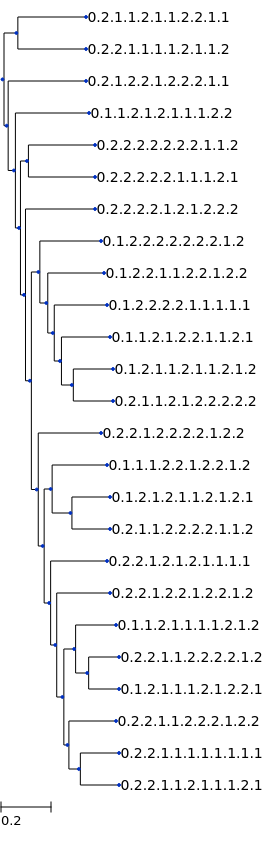In :
jc_corrected_hamming_tree = tree_from_distance_matrix(jc_correct_dm(hamming_dm), metric='upgma')
ete3.Tree(str(jc_corrected_hamming_tree), format=1).render("%%inline", tree_style=ts)

Out: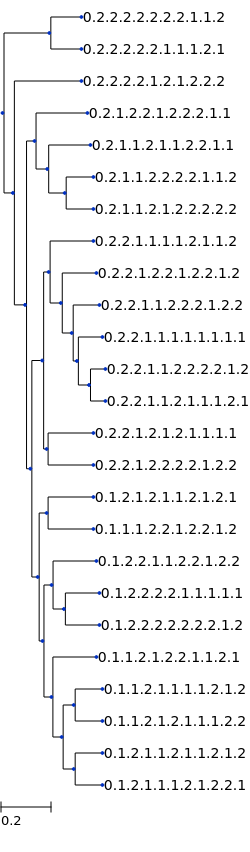#### 2.4.6.5.2 Understanding the name¶

As mentioned above, UPGMA has a rather complex sounding name: Unweighted Pair Group metric with Arithmetic mean. The Unweighted term indicates that all tip-to-tip distances contribute equally to each average that is computed (no weighted averages are being computed). The Pair Group term implies that all internal nodes, including the root node, will be strictly bifurcating, or descent to exactly two other nodes (either internal or terminal). The Arithmetic mean term implies that distances to each clade are the mean of distances to all members of that clade.

NOTE: The text below here gets very rough as these sections are currently being written or re-written.

### 2.4.6.6 Phylogenetic reconstruction with neighbor-joining¶

This section is currently a placeholder. You can track progress on this section through issue #119. In the meantime, I recommend Chapter 5.2.2 of The Phylogenetic Handbook, by Lemey, Salemi, and Vandamme for discussion of this topic. You can also refer to the scikit-bio implementation of Neighbor Joining, which will be used here (the source code is linked from that page).

One invalid assumption that is made by UPGMA is inherent in Step 1, where each branch connecting the internal node to a tip is set to half of the length between the tips. This assumes the mutation rates are constant throughout the tree, or in other words that the tree is ultrametric. This is not likely to be the case in the real world, as different lineages in the tree might be undergoing different selective pressures, leading to different rates of evolution. Neighboring joining is a distance-based phylogenetic reconstruction approach that does not assume ultrametricity.

In :
nj_tree = tree_from_distance_matrix(jc_correct_dm(hamming_dm), metric='nj')
ete3.Tree(str(nj_tree), format=1).render("%%inline", tree_style=ts)

Out: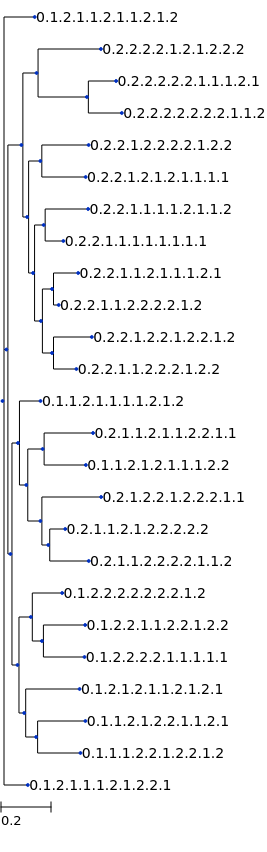### 2.4.6.7 Limitations of distance-based approaches¶

This section is currently a placeholder. You can track progress on this section through issue #119.

## 2.4.7 Bootstrap analysis¶

This section is currently a placeholder. You can track progress on this section through issue #119. In the meantime, I recommend Chapter 12.4.1 of The Phylogenetic Handbook, by Lemey, Salemi, and Vandamme for discussion of this topic.

## 2.4.8 Parsimony-based approaches to phylogenetic reconstruction¶

This section is currently a placeholder. You can track progress on this section through issue #119. In the meantime, I recommend Chapter 8 of The Phylogenetic Handbook, by Lemey, Salemi, and Vandamme for discussion of this topic.

### 2.4.8.1 How many possible phylogenies are there for a given collection of sequences?¶

This section is currently a placeholder. You can track progress on this section through issue #119. In the meantime, I recommend Chapter 3 of Inferring Phylogenies, the definitive text on this topic.

## 2.4.9 Statistical approaches to phylogenetic reconstruction¶

Table of Contents

### 2.4.9.1 Bayesian methods¶

This section is currently a placeholder. You can track progress on this section through issue #119. In the meantime, I recommend Chapter 7 of The Phylogenetic Handbook, by Lemey, Salemi, and Vandamme for discussion of this topic.

### 2.4.9.2 Maximum likelihood methods¶

This section is currently a placeholder. You can track progress on this section through issue #119. In the meantime, I recommend Chapter 6 of The Phylogenetic Handbook, by Lemey, Salemi, and Vandamme for discussion of this topic.

## 2.4.10 Rooted versus unrooted trees¶

The following is a rooted tree, which means that it includes an assumption about the last common ancestor of all sequences represented in the tree.An unrooted tree, like the following, doesn't include an assumption about the last common ancestor of all sequences:## 2.4.11 Acknowledgements¶

The material in this section was compiled while consulting the following sources:

1. The Phylogenetic Handbook (Lemey, Salemi, Vandamme)
2. Inferring Phylogeny (Felsenstein)
3. Richard Edwards's teaching website